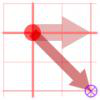Search by Topic

Resources tagged with Complex numbers similar to Farhan's Poor Square:

Filter by: Content type:
Age range:
Challenge level:

There are 6 results

Broad Topics > Numbers and the Number System > Complex numbers8 Methods for Three by One

Age 14 to 18 Challenge Level:

This problem in geometry has been solved in no less than EIGHT ways by a pair of students. How would you solve it? How many of their solutions can you follow? How are they the same or different?. . . .Twizzles Venture Forth

Age 14 to 16 Challenge Level:

Where we follow twizzles to places that no number has been before.Twizzle Twists

Age 14 to 16 Challenge Level:

Make the twizzle twist on its spot and so work out the hidden link.Twizzle Wind Up

Age 14 to 16 Challenge Level:

A loopy exploration of z^2+1=0 (z squared plus one) with an eye on winding numbers. Try not to get dizzy!What Are Numbers?

Age 7 to 18

Ranging from kindergarten mathematics to the fringe of research this informal article paints the big picture of number in a non technical way suitable for primary teachers and older students.Napoleon's Theorem

Age 14 to 18 Challenge Level:

Triangle ABC has equilateral triangles drawn on its edges. Points P, Q and R are the centres of the equilateral triangles. What can you prove about the triangle PQR?